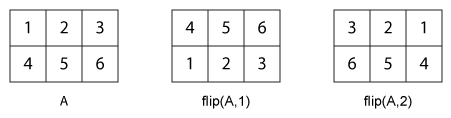flip

Flip order of elements

Description

example

B = flip(A) returns array B the same size as A, but with the order of the elements reversed. The dimension that is reordered in B depends on the shape of A:

• If A is vector, then flip(A) reverses the order of the elements along the length of the vector.

• If A is a matrix, then flip(A) reverses the elements in each column.

• If A is an N-D array, then flip(A) operates on the first dimension of A in which the size value is not 1.

example

B = flip(A,dim) reverses the order of the elements in A along dimension dim. For example, if A is a matrix, then flip(A,1) reverses the elements in each column, and flip(A,2) reverses the elements in each row.

Examples

collapse all

A = 'no word, no bond, row on.';
B = flip(A)
B =
'.no wor ,dnob on ,drow on'
A = [1;2;3];
B = flip(A)
B = 3×1

3
2
1

Create a diagonal matrix, A.

A = diag([100 200 300])
A = 3×3

100     0     0
0   200     0
0     0   300

Flip A without specifying the dim argument.

B = flip(A)
B = 3×3

0     0   300
0   200     0
100     0     0

Now, flip A along the second dimension.

B = flip(A,2)
B = 3×3

0     0   100
0   200     0
300     0     0

Create a 1-by-3-by-2 array.

A = zeros(1,3,2);
A(:,:,1) = [1 2 3];
A(:,:,2) = [4 5 6];
A
A =
A(:,:,1) =

1     2     3

A(:,:,2) =

4     5     6

Flip A without specifying the dim argument.

B = flip(A)
B =
B(:,:,1) =

3     2     1

B(:,:,2) =

6     5     4

Now, flip A along the third dimension.

B = flip(A,3)
B =
B(:,:,1) =

4     5     6

B(:,:,2) =

1     2     3

Create a 3-by-2 cell array.

A = {'foo',1000; 999,true; 'aaa','bbb'}
A=3×2 cell array
{'foo'}    {}
{}    {[   1]}
{'aaa'}    {'bbb' }

Flip A without specifying the dim argument.

B = flip(A)
B=3×2 cell array
{'aaa'}    {'bbb' }
{}    {[   1]}
{'foo'}    {}

Now, flip A along the second dimension.

B = flip(A,2)
B=3×2 cell array
{}    {'foo'}
{[   1]}    {}
{'bbb' }    {'aaa'}

Input Arguments

collapse all

Input array, specified as a vector, matrix, multidimensional array, table, or timetable.

Data Types: double | single | int8 | int16 | int32 | int64 | uint8 | uint16 | uint32 | uint64 | logical | char | string | struct | cell | table | timetable | categorical | datetime | duration | calendarDuration

Example: [1 2 3 4]

Example: ['abcde']

Example: [1 2; 3 4]

Example: {'abcde',[1 2 3]}

Example: table(rand(1,5),rand(1,5))

Dimension to operate along, specified as a positive integer scalar. If no value is specified, then the default is the first array dimension whose size does not equal 1.

The following illustration shows the difference between dim=1 and dim=2 when A is a matrix.